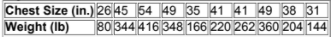×
Get Full Access to Elementary Statistics - 12 Edition - Chapter 4.8 - Problem 6cre
Get Full Access to Elementary Statistics - 12 Edition - Chapter 4.8 - Problem 6cre

×

# Scatterplot of Bear Chest Sizes and Weights Given belowISBN: 9780321836960 18

## Solution for problem 6CRE Chapter 4.8

Elementary Statistics | 12th Edition

• Textbook Solutions
• 2901 Step-by-step solutions solved by professors and subject experts
• Get 24/7 help from StudySoup virtual teaching assistantsElementary Statistics | 12th Edition

4 5 1 303 Reviews
23
0
Problem 6CRE

Scatterplot of Bear Chest Sizes and Weights. Given below are the chest sizes (inches) and weights (pounds) of 10 bears taken from Data Set 7 in Appendix. Construct a scatterplot. Based on the scatterplot, does there appear to be a correlation between the chest sizes of bears and their weightsStep-by-Step Solution:
Step 1 of 3

Solution  6CREStep 2 of 3

Step 3 of 3

##### ISBN: 9780321836960

Since the solution to 6CRE from 4.8 chapter was answered, more than 417 students have viewed the full step-by-step answer. This textbook survival guide was created for the textbook: Elementary Statistics, edition: 12. Elementary Statistics was written by and is associated to the ISBN: 9780321836960. The answer to “?Scatterplot of Bear Chest Sizes and Weights. Given below are the chest sizes (inches) and weights (pounds) of 10 bears taken from Data Set 7 in Appendix . Construct a scatterplot. Based on the scatterplot, does there appear to be a correlation between the chest sizes of bears and their weights” is broken down into a number of easy to follow steps, and 51 words. The full step-by-step solution to problem: 6CRE from chapter: 4.8 was answered by , our top Statistics solution expert on 03/15/17, 10:30PM. This full solution covers the following key subjects: weights, sizes, chest, scatterplot, bears. This expansive textbook survival guide covers 121 chapters, and 3629 solutions.

## Discover and learn what students are asking

Calculus: Early Transcendental Functions : Increasing and Decreasing Functions and the First DerivativeTest
?Using a Graph In Exercises 1 and 2, use the graph of to find (a) the largest open interval on which f is increasing, and (b) the largest open interval

Calculus: Early Transcendental Functions : Increasing and Decreasing Functions and the First DerivativeTest
?Using a Graph In Exercises 3-8, use the graph to estimate the open intervals on which the function is increasing or decreasing. Then find the open int

Statistics: Informed Decisions Using Data : Organizing Qualitative Data
?What is a bar graph? What is a Pareto chart?

Statistics: Informed Decisions Using Data : Comparing Three or More Means (One-Way Analysis of Variance)
?In Problems 7 and 8, fill in the ANOVA table.

Unlock Textbook Solution

Enter your email below to unlock your verified solution to:

Scatterplot of Bear Chest Sizes and Weights Given below